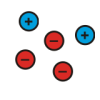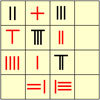Search by Topic

Resources tagged with Positive-negative numbers similar to Pair Sums:

Filter by: Content type:
Age range:
Challenge level:

There are 18 results

Broad Topics > Numbers and the Number System > Positive-negative numbersPair Sums

Age 11 to 14 Challenge Level:

Five numbers added together in pairs produce: 0, 2, 4, 4, 6, 8, 9, 11, 13, 15 What are the five numbers?Weights

Age 11 to 14 Challenge Level:

Different combinations of the weights available allow you to make different totals. Which totals can you make?Consecutive Numbers

Age 7 to 14 Challenge Level:

An investigation involving adding and subtracting sets of consecutive numbers. Lots to find out, lots to explore.Consecutive Negative Numbers

Age 11 to 14 Challenge Level:

Do you notice anything about the solutions when you add and/or subtract consecutive negative numbers?First Connect Three

Age 7 to 14 Challenge Level:

Add or subtract the two numbers on the spinners and try to complete a row of three. Are there some numbers that are good to aim for?Up, Down, Flying Around

Age 11 to 14 Challenge Level:

Play this game to learn about adding and subtracting positive and negative numbersMissing Multipliers

Age 7 to 14 Challenge Level:

What is the smallest number of answers you need to reveal in order to work out the missing headers?Strange Bank Account

Age 11 to 14 Challenge Level:

Imagine a very strange bank account where you are only allowed to do two things...Strange Bank Account (part 2)

Age 11 to 14 Challenge Level:

Investigate different ways of making £5 at Charlie's bank.Making Sense of Positives and Negatives

Age 11 to 14

This article suggests some ways of making sense of calculations involving positive and negative numbers.Adding and Subtracting Positive and Negative Numbers

Age 11 to 14

How can we help students make sense of addition and subtraction of negative numbers?Negative Numbers

Age 11 to 14

A brief history of negative numbers throughout the agesConnect Three

Age 11 to 16 Challenge Level:

In this game the winner is the first to complete a row of three. Are some squares easier to land on than others?Minus One Two Three

Age 14 to 16 Challenge Level:

Substitute -1, -2 or -3, into an algebraic expression and you'll get three results. Is it possible to tell in advance which of those three will be the largest ?Vector Racer

Age 11 to 16 Challenge Level:

The classic vector racing game.Negatively Triangular

Age 14 to 16 Challenge Level:

How many intersections do you expect from four straight lines ? Which three lines enclose a triangle with negative co-ordinates for every point ?The History of Negative Numbers

Age 11 to 18

This article -useful for teachers and learners - gives a short account of the history of negative numbers.Vector Gem Collector

Age 14 to 18 Challenge Level:

Use vectors to collect as many gems as you can and bring them safely home!